SNAP Library 6.0, Developer Reference  2020-12-09 16:24:20 SNAP, a general purpose, high performance system for analysis and manipulation of large networks
TUndirNet::TEdgeI Class Reference

Edge iterator. Only forward iteration (operator++) is supported. More...

`#include <network.h>`

Collaboration diagram for TUndirNet::TEdgeI: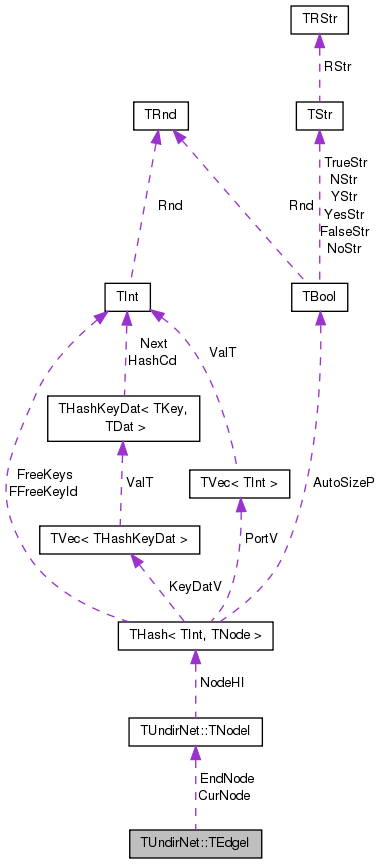[legend]

## Public Member Functions

TEdgeI ()

TEdgeI (const TNodeI &NodeI, const TNodeI &EndNodeI, const int &EdgeN=0)

TEdgeI (const TEdgeI &EdgeI)

TEdgeIoperator= (const TEdgeI &EdgeI)

TEdgeIoperator++ (int)
Increment iterator. More...

bool operator< (const TEdgeI &EdgeI) const

bool operator== (const TEdgeI &EdgeI) const

int GetId () const
Returns edge ID. Always returns -1 since only edges in multigraphs have explicit IDs. More...

int GetSrcNId () const
Returns the source of the edge. Since the network is undirected, this is the node with a smaller ID of the edge endpoints. More...

int GetDstNId () const
Returns the destination of the edge. Since the network is undirected, this is the node with a greater ID of the edge endpoints. More...

TNodeI CurNode

TNodeI EndNode

int CurEdge

class TUndirNet

## Detailed Description

Edge iterator. Only forward iteration (operator++) is supported.

Definition at line 3486 of file network.h.

## Constructor & Destructor Documentation

 TUndirNet::TEdgeI::TEdgeI ( )
inline

Definition at line 3491 of file network.h.

3491 : CurNode(), EndNode(), CurEdge(0) { }
 TUndirNet::TEdgeI::TEdgeI ( const TNodeI & NodeI, const TNodeI & EndNodeI, const int & EdgeN = `0` )
inline

Definition at line 3492 of file network.h.

3492 : CurNode(NodeI), EndNode(EndNodeI), CurEdge(EdgeN) { }
 TUndirNet::TEdgeI::TEdgeI ( const TEdgeI & EdgeI )
inline

Definition at line 3493 of file network.h.

3493 : CurNode(EdgeI.CurNode), EndNode(EdgeI.EndNode), CurEdge(EdgeI.CurEdge) { }

## Member Function Documentation

 int TUndirNet::TEdgeI::GetDstNId ( ) const
inline

Returns the destination of the edge. Since the network is undirected, this is the node with a greater ID of the edge endpoints.

Definition at line 3504 of file network.h.

References TUndirNet::TNodeI::GetOutNId().

3504 { return CurNode.GetOutNId(CurEdge); }
int GetOutNId(const int &NodeN) const
Returns ID of NodeN-th out-node (the node the current node points to).
Definition: network.h:3471

Here is the call graph for this function: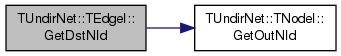Here is the caller graph for this function: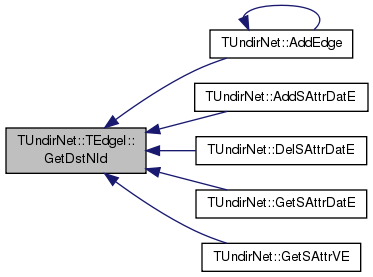int TUndirNet::TEdgeI::GetId ( ) const
inline

Returns edge ID. Always returns -1 since only edges in multigraphs have explicit IDs.

Definition at line 3500 of file network.h.

3500 { return -1; }
 int TUndirNet::TEdgeI::GetSrcNId ( ) const
inline

Returns the source of the edge. Since the network is undirected, this is the node with a smaller ID of the edge endpoints.

Definition at line 3502 of file network.h.

References TUndirNet::TNodeI::GetId().

3502 { return CurNode.GetId(); }
int GetId() const
Returns ID of the current node.
Definition: network.h:3453

Here is the call graph for this function: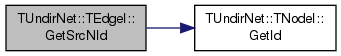Here is the caller graph for this function: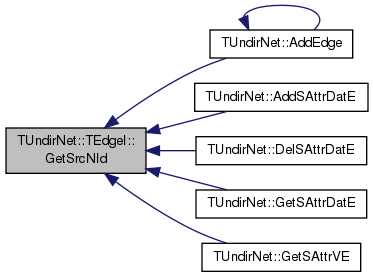TEdgeI& TUndirNet::TEdgeI::operator++ ( int )
inline

Increment iterator.

Definition at line 3496 of file network.h.

References TUndirNet::TNodeI::GetOutDeg().

3496 { do { CurEdge++; if (CurEdge >= CurNode.GetOutDeg()) { CurEdge=0; CurNode++; while (CurNode < EndNode && CurNode.GetOutDeg()==0) { CurNode++; } } } while (CurNode < EndNode && GetSrcNId()>GetDstNId()); return *this; }
int GetOutDeg() const
Returns out-degree of the current node (returns same as value GetDeg() since the network is undirecte...
Definition: network.h:3459
int GetDstNId() const
Returns the destination of the edge. Since the network is undirected, this is the node with a greater...
Definition: network.h:3504
int GetSrcNId() const
Returns the source of the edge. Since the network is undirected, this is the node with a smaller ID o...
Definition: network.h:3502

Here is the call graph for this function: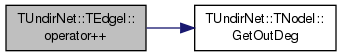bool TUndirNet::TEdgeI::operator< ( const TEdgeI & EdgeI ) const
inline

Definition at line 3497 of file network.h.

References CurEdge, and CurNode.

3497 { return CurNode<EdgeI.CurNode || (CurNode==EdgeI.CurNode && CurEdge<EdgeI.CurEdge); }
 TEdgeI& TUndirNet::TEdgeI::operator= ( const TEdgeI & EdgeI )
inline

Definition at line 3494 of file network.h.

References CurEdge, CurNode, and EndNode.

3494 { if (this!=&EdgeI) { CurNode=EdgeI.CurNode; EndNode=EdgeI.EndNode; CurEdge=EdgeI.CurEdge; } return *this; }
 bool TUndirNet::TEdgeI::operator== ( const TEdgeI & EdgeI ) const
inline

Definition at line 3498 of file network.h.

References CurEdge, and CurNode.

3498 { return CurNode == EdgeI.CurNode && CurEdge == EdgeI.CurEdge; }

## Friends And Related Function Documentation

 friend class TUndirNet
friend

Definition at line 3505 of file network.h.

## Member Data Documentation

 int TUndirNet::TEdgeI::CurEdge
private

Definition at line 3489 of file network.h.

Referenced by operator<(), operator=(), and operator==().

 TNodeI TUndirNet::TEdgeI::CurNode
private

Definition at line 3488 of file network.h.

Referenced by operator<(), operator=(), and operator==().

 TNodeI TUndirNet::TEdgeI::EndNode
private

Definition at line 3488 of file network.h.

Referenced by operator=().

The documentation for this class was generated from the following file: